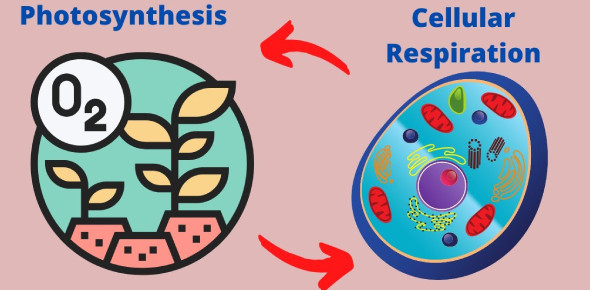# Photosynthesis And Respiration Quiz: Trivia!

14 Questions | Attempts: 235
ShareSettings.

• 1.
What does photosynthesis use to convert water and carbon dioxide into oxygen and high energy sugars?
• A.

ATP energy

• B.

• C.

Sunlight energy

• D.

• 2.
What does respiration produce?
• A.

Water and oxygen

• B.

Glucose and sugar

• C.

Carbon dioxide and awter

• D.

: oxygen and glucose

• 3.
What does respiration do when it breaks down from molecules in the presents of oxygen?
• A.

Release energy

• B.

Absorb energy

• C.

Produce glucose

• D.

All the above

• 4.
What is the equation for respiration?
• A.

6O2+6CH12O6 > 6CO2+6H2O+energy

• B.

6CO2+6H2O > C6H12O6+6O2

• C.

C6H12O6+6O2 > 6O2+6CH12O6

• D.

6CO2+6H2O+energy > 6CO2+6H2O

• 5.
What is the equation for photosynthesis?
• A.

6O2+6CH12O6 > 6CO2+6H2O+energy

• B.

6CO2+6H2O > C6H12O6+6O2

• C.

6CO2+6H2O+energy > 6CO2+6H2O

• D.

C6H12O6+6O2 > 6O2+6CH12O6

• 6.
During the process of photosynthesis, what happens?
• A.

Chemical energy is converted to light energy

• B.

Produce GTP

• C.

Light energy is converted to chemical energy

• D.

Essential to oxygen production

• 7.
In which organelle does photosynthesis take place?
• A.

Chlorophyll

• B.

Thylakoid

• C.

Chloroplast

• D.

Impalas

• 8.
What is the one product of photosynthesis?
• A.

Oxygen

• B.

Glucose

• C.

Hydogen

• D.

Carbon dioxide

• 9.
What is the difference between aerobic respiration and anaerobic respiration?
• A.

Aerobic is the process that requires oxygen and anaerobic respiration doesn’t.

• B.

Anaerobic is the process that requires oxygen and areobic respiration doesn't.

• C.

Aerobic is the process that requires glucose and anaerobic respiration doesn't.

• D.

Anaerobic is the process that requires glucose and anaerobic respiration doesn't.

• 10.
Why is light and chlorophyll needed for photosynthesis?
• A.

To lower the energy level

• B.

To raises the energy level

• C.

To equal the energy level

• D.

To store energy

• 11.
What happens in a light-dependent reaction?
• A.

• B.

It produces carbon dioxide, glucose, and convert ATP and NADPH into the energy carriers ADP and NADP.

• C.

• D.

It produces carbon dioxide, glucose, and convert ADP and NADP into the energy carriers ATP and NADPH.

• 12.
The light-independent reaction of photosynthesis is also known as the?
• A.

Priestly cycle

• B.

Van Helmont cycle

• C.

Calvin Cycle

• D.

Igenhousz cycle

• 13.
Aerobic respiration produce substantially more ATP than anaerobic respiration.
• A.

True

• B.

False

• 14.
What happens during the Calvin Cycle?
• A.

It uses ATP and NADPH from the light-dependent reactions to produce low-energy sugars.

• B.

It uses ATP and NADPH from the light-independent reactions to produce low-energy sugars.

• C.

It uses ATP and NADPH from the light-dependent reactions to produce high-energy sugars.

• D.

It uses ATP and NADPH from the light-independent reactions to produce high-energy sugars.

## Related TopicsBack to top
×

Wait!
Here's an interesting quiz for you.